# Camera Case & Pads of Paper Weigh In

## 3 Act Math With Partial Variation Linear Relations

Here’s another task that is a good next step once you’ve tackled the Tech Weigh In 3 act math task that covers direct and partial variation linear relations. This task gives students another chance to get some exposure to partial variation linear relations and could provide an opportunity for the teacher to begin consolidating the topic by moving towards some more abstract concepts like the slope formula and finding linear equations given two points. Similar tasks to check out would be Stacking Paper, Stacking Paper Sequel and Thick Stacks.

Special thanks to Justin Levack again for coming up with the “weigh in” idea and pushing me to actually record this with him when we are all running out of steam at the end of the school year.

## Act 1 – Introduce the Problem

As stated above, I’d suggest tackling the Tech Weigh In 3 act math task prior to doing this task. Because I would do Tech Weigh In first, I feel that an act 1 is not really necessary for this particular problem. Tech Weigh In sets the stage nicely and this is more of a sequel than anything else. This photo would likely take care of it: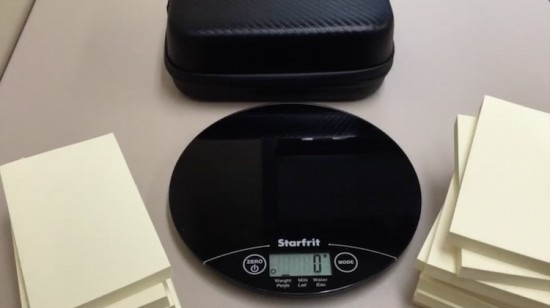What’s the question?

As always, get your students talking about what they think this math task is going to focus on. Writing the questions out is always helpful. In this case, since they’ve already done Tech Weigh In, my students are pretty confident we’re going to be finding out how much the camera case weighs (or initial value). However, I use this task to get a bit more specific and focus on questions along these lines:

• How much does the camera case weigh and what do we call this number? (i.e.: initial value)
• How much does each pad of paper weigh and what do we call this number? (i.e.: slope/rate of change)
• What is an equation that could represent this linear relation?
• What would the weight be if we stacked _____ pads of paper?
• How many pads of paper would it take to get a weight of ____?

Making predictions is always a good practice after some questions have been shared out.

## Act 2 – Giving Some Information## Exemplars

Here’s two exemplars of how students may approach this through an inquiry approach: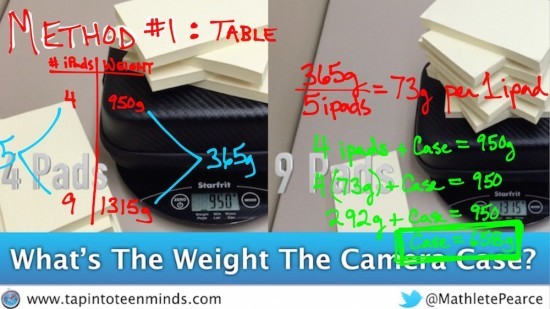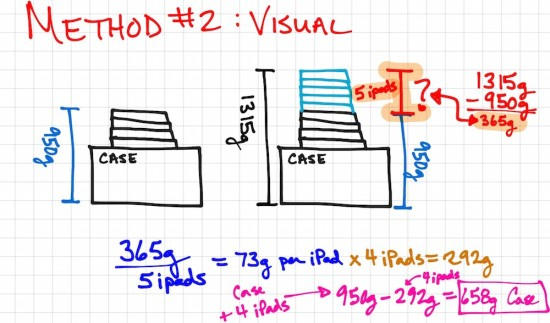Many teachers might consider using a procedure to find the equation of a line given two points using the slope formula as the next logical step. However, I have witnessed a few students essentially use substitution to solve this type of problem. It might not look as organized as how students are instructed to solve a system of linear equations, but the thinking is much the same. Here’s an example of what it might look like (but this is probably too organized for a real case):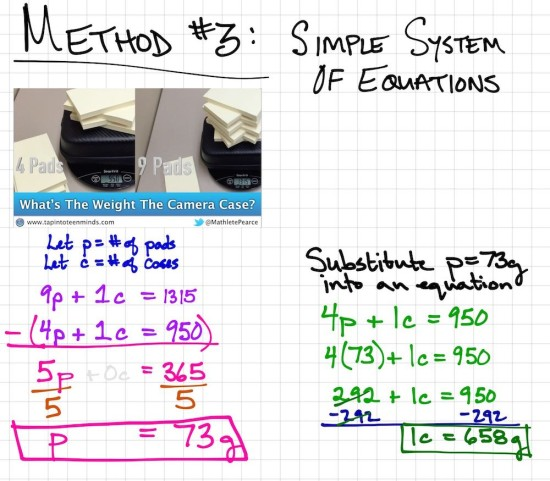I’d suggest that after solving a simple system of equations using substitution, you might want to move towards consolidating the exemplars above to the slope formula and solving y = mx + b algebraically for the initial value, b: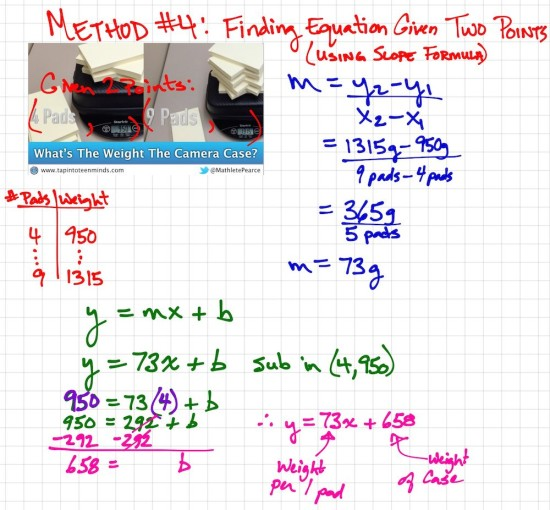## Access Resources

Check out all of the resources here. Or, you can grab what you want individually below:

## Student Exemplars

Have you tried the task? Let us know how to make it better in the comments!

## New to Using 3 Act Math Tasks?

Download the 2-page printable 3 Act Math Tip Sheet to ensure that you have the best start to your journey using 3 Act math Tasks to spark curiosity and fuel sense making in your math classroom!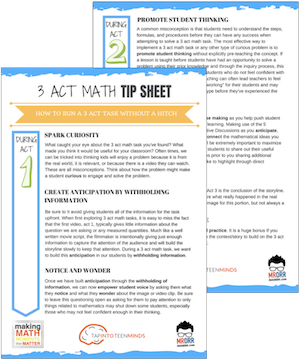## Share With Your Learning Community:I’m Kyle Pearce and I am a former high school math teacher. I’m now the K-12 Mathematics Consultant with the Greater Essex County District School Board, where I uncover creative ways to spark curiosity and fuel sense making in mathematics. Read more.

Access Other Real World Math Tasks

## Search More 3 Act Math Tasks

Spark Curiosity!